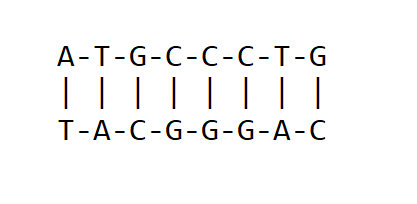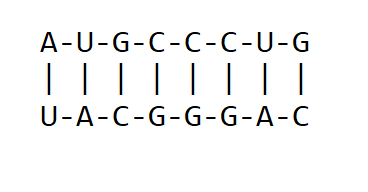# Reverse complement of DNA strand using Python

In this article, we will cover, how to Reverse the complement of DNA or RNA sequences in Python.

Example:

```DNA strand: ATGCCGAGCA
Complementary Strand: TACGGCTCGT
Reverse-Complementary strand: ACGAGCCGTA```

## An overview of DNA and RNA as used in Molecular Biology

The genetic material of living organisms is made up of Deoxyribonucleic acid(DNA) or Ribonucleic acid (RNA). The primary structure of DNA and RNA is made up of a sequence of nucleotide bases. The structure of DNA can be a double-stranded or single-stranded sequence of nucleotides(bases). For double-stranded nucleic acids, the nucleotide bases pair in a given rule which is unique to DNA and RNA. For DNA, there exist four types of bases namely; Adenine(A), Thymine(T), Guanine(G), and Cytosine(C).  Therefore, DNA can be identified as containing ATGC bases. The pairing of bases in DNA  is that Adenine pairs with Thymine(with a double bond) while Guanine Pairs with Cytosine (with a triple bond). i.e A=T and G≡C as shown below.DNA base pairing. The upper strand is complementary to the downer strand and vice versa

For RNA, all instances of Thymine are replaced by Uracil. This means that for double-stranded RNA, Adenine pairs with Uracil while Guanine pairs with Cytosine A=U and G≡C as shown below:RNA base pairing. Each strand is a complementary sequence to one another

## Reverse Complement of a DNA or RNA

A Reverse Complement converts  RNA or DNA sequence into its reverse, complement counterpart. One of the major questions in Molecular Biology to solve using computational approaches is to find the reverse complement of a sequence. This is always done so to work with the reversed-complement of a  given sequence if it contains an open reading frame(a region that encodes for a protein sequence during the transcription process) on the reverse strand.  One could be interested to verify that the sequence is a DNA or RNA before finding its reverse complement

### How to identify if the sequences of DNA and RNA

One of the major tasks in Bioinformatics in computational molecular biology and bioinformatics is to verify if the sequence is DNA or RNA. To do this we can use the set method to verify a sequence.

## Method 1:  Verify if a sequence is DNA and RNA

Step 1:

In the set method, we convert the input sequence into a set. We combine the set obtained with a reference DNA set(ATGC) or RNA set(AUGC) using the union function of the set. This is done so that the input sequence is rendered valid even if it does not contain all four types of nucleotide bases. For instance, TTTTTTTAAA is a valid DNA even though it contains only two types of bases. Also, UUUUUUUUGGG is a valid RNA.

## Python3

 `def` `verify(sequence):` `    ``'''This code verifies if a sequence is a DNA or RNA'''` `    ``# set the input sequence` `    ``seq ``=` `set``(sequence)` `    `  `    ``# confirm if its elements is equal to the` `    ``# set of valid DNA bases` `    ``# Use a union method to ensure the sequence is` `    ``# verified if does not contain all the bases` `    ``if` `seq ``=``=` `{``"A"``, ``"T"``, ``"C"``, ``"G"``}.union(seq):` `        ``return` `"DNA"` `    ``elif` `seq ``=``=` `{``"A"``, ``"U"``, ``"C"``, ``"G"``}.union(seq):` `        ``return` `"RNA"` `    ``else``:` `        ``return` `"Invalid sequence"`     `seq1 ``=` `"ATGCAGCTGTGTTACGCGAT"` `seq2 ``=` `"UGGCGGAUAAGCGCA"` `seq3 ``=` `"TYHGGHHHHH"`   `print``(seq1 ``+` `" is "` `+` `verify(seq1))` `print``(seq2 ``+` `" is "` `+` `verify(seq2))` `print``(seq3 ``+` `" is "` `+` `verify(seq3))`

Output:

```ATGCAGCTGTGTTACGCGAT is DNA
UGGCGGAUAAGCGCA is RNA
TYHGGHHHHH is Invalid sequence```

Step 2:

This function returns a reverse complement of a DNA or RNA strand.

## Python3

 `def` `verify(sequence):` `    ``'''This code verifies if a sequence is a DNA or RNA'''` `    `  `    ``# set the input sequence` `    ``seq ``=` `set``(sequence)` `    `  `    ``# confirm if its elements is equal to ` `    ``# the set of valid DNA bases` `    ``# Use a union method to ensure the` `    ``# sequence is verified if does not` `    ``# contain all the bases` `    ``if` `seq ``=``=` `{``"A"``, ``"T"``, ``"C"``, ``"G"``}.union(seq):` `        ``return` `"DNA"` `    ``elif` `seq ``=``=` `{``"A"``, ``"U"``, ``"C"``, ``"G"``}.union(seq):` `        ``return` `"RNA"` `    ``else``:` `        ``return` `"Invalid sequence"`     `def` `rev_comp_st(seq):` `    ``'''This function returns a reverse complement ` `    ``of a DNA or RNA strand'''` `    ``verified ``=` `verify(seq)` `    ``if` `verified ``=``=` `"DNA"``:` `      `  `        ``# complement strand` `        ``seq ``=` `seq.replace(``"A"``, ``"t"``).replace(` `            ``"C"``, ``"g"``).replace(``"T"``, ``"a"``).replace(``"G"``, ``"c"``)` `        ``seq ``=` `seq.upper()` `        `  `        ``# reverse strand` `        ``seq ``=` `seq[::``-``1``]` `        ``return` `seq`   `    ``elif` `verified ``=``=` `"RNA"``:` `      `  `        ``# complement strand` `        ``seq ``=` `seq.replace(``"A"``, ``"u"``).replace(` `            ``"C"``, ``"g"``).replace(``"U"``, ``"a"``).replace(``"G"``, ``"c"``)` `        ``seq ``=` `seq.upper()` `        `  `        ``# reverse strand` `        ``seq ``=` `seq[::``-``1``]` `        ``return` `seq` `    ``else``:` `        ``return` `"Invalid sequence"`     `# test variables` `seq1 ``=` `"ATGCAGCTGTGTTACGCGAT"` `seq2 ``=` `"UGGCGGAUAAGCGCA"` `seq3 ``=` `"TYHGGHHHHH"`   `print``(``"The reverse complementary strand of "` `+` `      ``seq1 ``+` `" is "` `+` `rev_comp_st(seq1))` `print``(``"The reverse complementary strand of "` `+` `      ``seq2 ``+` `" is "` `+` `rev_comp_st(seq2))` `print``(``"The reverse complementary strand of "` `+` `      ``seq3 ``+` `" is "` `+` `rev_comp_st(seq3))`

Output:

The reverse complementary strand of ATGCAGCTGTGTTACGCGAT is ATCGCGTAACACAGCTGCAT

The reverse complementary strand of UGGCGGAUAAGCGCA is UGCGCUUAUCCGCCA

The reverse complementary strand of TYHGGHHHHH is Invalid sequence

## Method 2:  Use of if statement

Another method of finding a complementary sequence of DNA or RNA is the use of if statements. The sequence is first verified if it is DNA or RNA. If a sequence is DNA, All instances of A are replaced by T, all instances of T are replaced by A, all instances of G are replaced by C and all instances of C are replaced by G.

## Python3

 `def` `verify(sequence):` `    ``'''This code verifies if a sequence is a DNA or RNA'''` `    `  `    ``# set the input sequence` `    ``seq ``=` `set``(sequence)` `    `  `    ``# confirm if its elements is equal to ` `    ``# the set of valid DNA bases` `    ``# Use a union method to ensure the` `    ``# sequence is verified if does not` `    ``# contain all the bases` `    ``if` `seq ``=``=` `{``"A"``, ``"T"``, ``"C"``, ``"G"``}.union(seq):` `        ``return` `"DNA"` `    ``elif` `seq ``=``=` `{``"A"``, ``"U"``, ``"C"``, ``"G"``}.union(seq):` `        ``return` `"RNA"` `    ``else``:` `        ``return` `"Invalid sequence"`     `def` `rev_comp_if(seq):` `    ``comp ``=` `[]` `    ``if` `verify(seq) ``=``=` `"DNA"``:` `        ``for` `base ``in` `seq:` `            ``if` `base ``=``=` `"A"``:` `                ``comp.append(``"T"``)` `            ``elif` `base ``=``=` `"G"``:` `                ``comp.append(``"C"``)` `            ``elif` `base ``=``=` `"T"``:` `                ``comp.append(``"A"``)` `            ``elif` `base ``=``=` `"C"``:` `                ``comp.append(``"G"``)` `    ``elif` `verify(seq) ``=``=` `"RNA"``:` `        ``for` `base ``in` `seq:` `            ``if` `base ``=``=` `"U"``:` `                ``comp.append(``"A"``)` `            ``elif` `base ``=``=` `"G"``:` `                ``comp.append(``"C"``)` `            ``elif` `base ``=``=` `"A"``:` `                ``comp.append(``"U"``)` `            ``elif` `base ``=``=` `"C"``:` `                ``comp.append(``"G"``)` `    ``else``:` `        ``return` `"Invalid Sequence"` `      `  `    ``# reverse the sequence` `    ``comp_rev ``=` `comp[::``-``1``]` `    `  `    ``# convert list to string` `    ``comp_rev ``=` `"".join(comp_rev)` `    ``return` `comp_rev`     `seq1 ``=` `"ATGCAGCTGTGTTACGCGAT"` `seq2 ``=` `"UGGCGGAUAAGCGCA"` `seq3 ``=` `"TYHGGHHHHH"`   `print``(``"The reverse complementary strand of "` `+` `      ``seq1 ``+` `" is "` `+` `rev_comp_if(seq1))` `print``(``"The reverse complementary strand of "` `+` `      ``seq2 ``+` `" is "` `+` `rev_comp_if(seq2))` `print``(``"The reverse complementary strand of "` `+` `      ``seq3 ``+` `" is "` `+` `rev_comp_if(seq3))`

Output:

The reverse complementary strand of ATGCAGCTGTGTTACGCGAT is ATCGCGTAACACAGCTGCAT

The reverse complementary strand of UGGCGGAUAAGCGCA is UGCGCUUAUCCGCCA

The reverse complementary strand of TYHGGHHHHH is Invalid Sequence

Whether you're preparing for your first job interview or aiming to upskill in this ever-evolving tech landscape, GeeksforGeeks Courses are your key to success. We provide top-quality content at affordable prices, all geared towards accelerating your growth in a time-bound manner. Join the millions we've already empowered, and we're here to do the same for you. Don't miss out - check it out now!

Previous
Next